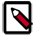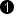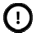## Operator ColorTransform

The operator ColorTransform transforms the RGB stream at the input into an RGB stream with weighted components. The transformation is performed by a 3x3 matrix multiplication with the input RGB vector.

The transformation matrix is defined by the parameter Coefficients. This parameter is a 3x3 matrix containing double precision coefficients. The following formula represents the transformation algorithm.

For calculation fixed point precision values are used. Parameters Precision and Resolution specify the multiplication precisions.

• Precision defines the number of bits used to encode the coefficients.

• Resolution defines the number of fractional bits of a coefficient.

For example a precision of 8 bit and a resolution of 6 bits will result in coefficients within the range .

Signed values are allowed as coefficients. When the field parameter Coefficients is set to dynamic (i.e., transformation coefficients can be altered during runtime), the output link is always signed. One of the precision bits is used as the signed bit.

The results of the matrix multiplication are provided. They are rounded to the next integer value. The output bit width is automatically determined from the input bit width and parameter settings.

Less Resources for Static ParameterThe operator requires less FPGA resources if the parameter Coefficients is set to static.

### I/O Properties

Property Value
Operator Type O
Input Link I, image data input
Output Link O, image data input

Bit Width [3, 54] unsigned, [6, 54] signed autoArithmetic {unsigned, signed} auto
Parallelism any as I
Kernel Columns 1 as I
Kernel Rows 1 as I
Img Protocol {VALT_IMAGE2D, VALT_LINE1D, VALT_PIXEL0D} as I
Color Format VAF_COLOR as I
Color Flavor FL_RGB as I
Max. Img Width any as I
Max. Img Height any as I
 The output bit width is automatically determined from the input bit width and the parameter settings.

### Parameters

Resolution
Type static parameter
Default 8
Range auto

Defines the number of fractional bits of a coefficient. The range depends on the input bit width and the value of parameter Precision. Mind the following limitations:

Resolution > 0

Precision > Resolution

Precision + Resolution <= 32

Precision
Type static parameter
Default 16
Range auto

Defines the number of bits to encode the whole coefficient - resolution bits and sign bit included. The range depends on the input bit width and the value of parameter Precision. Mind the following limitations:

Resolution > 0

Precision > Resolution

Precision + Resolution <= 32

Coefficients
Only Embedded VisualApplets (eVA): Deviating Parameter Interface during RuntimeDuring runtime on eVA platforms, this parameter shows another parameter interface than during design time in the VisualApplets GUI: Field parameter Coefficents is replaced by separate index parameters and value parameters (parameter names: CoefficientIndex and CoefficientValue). The value is written on access to CoefficientValue.